# Introduction to Scala Programming Language-Features & Future Scope

### What is Scala?

Scalable Language, Scala is a general-purpose programming language, both object-oriented and highly functional programming language. It is easy to learn, simple and aids programmers in writing codes in a simple, sophisticated and type-safe manner. It also enables developers and programmers to be more productive.

• Even though Scala is a relatively new language, it has garnered enough users and has wide community support – because it’s touted as the most user-friendly language.
• Scala is influenced by Java, Haskell, Lisp, Pizza, etc. and influenced to F#, Red, etc.
• The file extension of the Scala source file may be either. scala or.sc.
• You can create any kind of application like a web application, enterprise application, mobile application, desktop-based application, etc.

Scala Program Example:

input: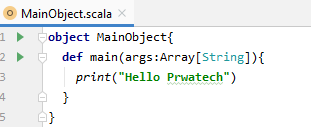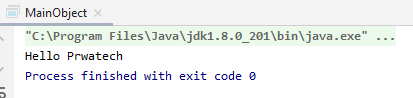## Prerequisites for Learning Scala

Scala is easy to learn the language has minimal prerequisites. If you are someone with a basic knowledge of C/C++, then you will be easily able to get started with Scala. Since Scala is developed on top of Java. The basic programming function in Scala is similar to Java. So, if you have some basic knowledge of Java syntax and OOPs concept, it would be helpful for you to work in Scala.

Introduction:

• Scala is a general-purpose programming language. It was created and developed by Martin Odersky. Martin started working on Scala in 2001 at the Ecole Polytechnique Federale de Lausanne (EPFL). It was officially released on January 20, 2004.
• Scala is not an extension of Java, but it is completely interoperable with it. While compilation, the Scala file translates to Java bytecode and runs on JVM (Java Virtual machine).
• Scala was designed to be both object-oriented and functional. It is a pure object-oriented language in the sense that every value is an object and functional language in the sense that every function is a value. The name of Scala is derived from word scalable which means it can grow with the demand of users.

### Why Scala?

Scala has many reasons for being popular among programmers. Few of the reasons are:

• Easy to Start: Scala is a high-level language so it is closer to other popular programming languages like Java, C, C++. Thus it becomes very easy to learn Scala for anyone. For Java programmers, Scala is more easy to learn.
• It contains the best Features: Scala contains the features of different languages like C, C++, Java, etc. which makes it more useful, scalable and productive.
• Close integration with Java: The source code of the Scala is designed in such a way that its compiler can interpret the Java classes. Also, its compiler can utilize the frameworks, Java Libraries, and tools, etc. After compilation, Scala programs can run on JVM.
• Web-Based & Desktop Application Development: For the web applications it provides support by compiling to JavaScript. Similarly, for desktop applications, it can be compiled to JVM bytecode.
• Used by Big Companies: Most of the popular companies like Apple, Twitter, Walmart, Google, etc. move their most of the codes to Scala from some other languages. reason being it is highly scalable and can be used in backend operations.

### Where we can use Scala?

• Web applications
• Utilities and libraries
• Data streaming with Akka
• Parallel batch processing
• Concurrency and distributed application
• Data analysis with Spark
• AWS lambda expression
• Ad hoc scripting in REPL etc.In Scala, you can create any type of application in less time and coding whether it is web-based, mobile-based or desktop-based application. Scala provides you powerful tools and API by using which you can create applications. Here, you can use a play framework which provides a platform to build web application rapidly.

## Comparison Between Scala and Java

There is a simple question that every developer should ask, why should he/she go for Scala instead of java?  The following comparison will help you make your decision:

 Java Scala Complex syntax Simple syntax Rewriting is needed Rewriting is not required Dynamic in nature Statically-typed No assurance of bug-free codes Assurance of lesser defects

Scala and Java are two of the most important programming languages in today’s world. Though there are a lot of similarities between the two, there are many more differences between them.

Scala, when compared to Java, is relatively a new language. It is a machine-compiled language, whereas Java is object-oriented.  Scala has enhanced code readability and conciseness. It has the facility to work in a multi-core architecture environment. The code that is written in Java can be written in Scala in half the number of lines.

### Comparison Between Scala and Python:

Python is a high level, interpreted and general-purpose dynamic programming language that focuses on code readability. Python requires less typing, provides new libraries, fast prototyping, and several other new features.
Scala is a high-level language.it is a purely object-oriented programming language. The source code of the Scala is designed in such a way that its compiler can interpret the Java classes.

### Below are some major differences between Python and Scala:

 PYTHON SCALA Python is a dynamically typed language. Scala is a statically typed language. We don’t need to specify objects in Python because it is a dynamically typed Object Oriented Programming language. We need to specify the type of variables and objects in Scala because Scala is statically typed Object Oriented Programming language. Python is easy to learn and use. Scala is less difficult to learn than Python. Extra work is created for the interpreter at the runtime. No extra work is created in Scala and thus it is 10 times faster than Python. The data types are decided by it during runtime. This is not the case in Scala that is why while dealing with large data process, Scala should be considered instead of Python Python’s Community is huge compared to Scala. Scala also has good community support. But still, it is lesser than Python.

## Features of Scala

• Object-oriented Programing Language: Scala is both a functional Programming Language & an object-oriented programming language. Every variable & value which is used in Scala is implicitly saved as an object by default.
• Extensible Programming Language: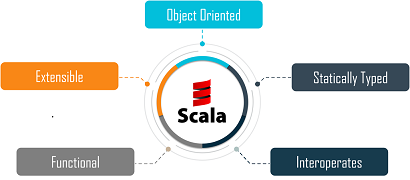Scala can support multiple language constructs without the need of any Domain Specific Language (DSL)Extensions, Libraries, and APIs.

• Statically Typed Programming Language: Scala language binds the Datatype to the variable in its entire scope.
• Functional Programming Language: Scala language provides a lightweight syntax for defining functions, it supports higher-order functions, it allows functions to be nested.
• Interoperability:

Scala compiles the code using the scala compiler and converts code into Java Byte Code and Executes it on JVM.

These were the Features of Scala language and let us get into a few of the frameworks of Scala that are capable to support.

Frameworks of Scala: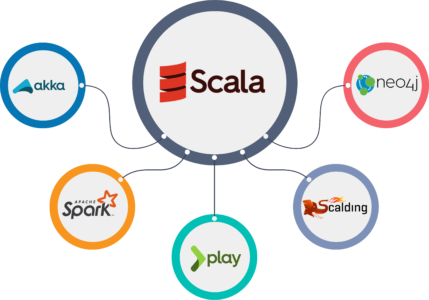Akka, Spark, Play, Neo4j, Scalding are some of the major frameworks that Scala language can support.

• Akka is a toolkit on runtime for building highly concurrent, distributed, and fault-tolerant applications on the JVM. Akka is written in Scala language, with language bindings provided for both Scala and Java.
• Spark Framework is designed to handle, and process big-data and it solely supports Scala language.
• Play framework is designed to create web applications and it uses Scala in the process in order to obtain the best in class performance.
• Scalding is a domain-specific language (DSL)in the Scala programming language, which integrates Cascading. It is a functional programming paradigm used in Scala which is much closer than Java to the original model for MapReduce functions.
• Neo4j is a java spring framework supported by Scala language with domain-specific functionality, analytical capabilities, graph algorithms, and many more.

These were the popular Frameworks supported by Scala.

### Scope for Scala

Scala is the miracle of the 20th century in multiple streams. It has seen astounding growth since day one and it is for sure it is one of the programming languages which is in higher demand. The stats below explain more about the scope of Scala in the near future.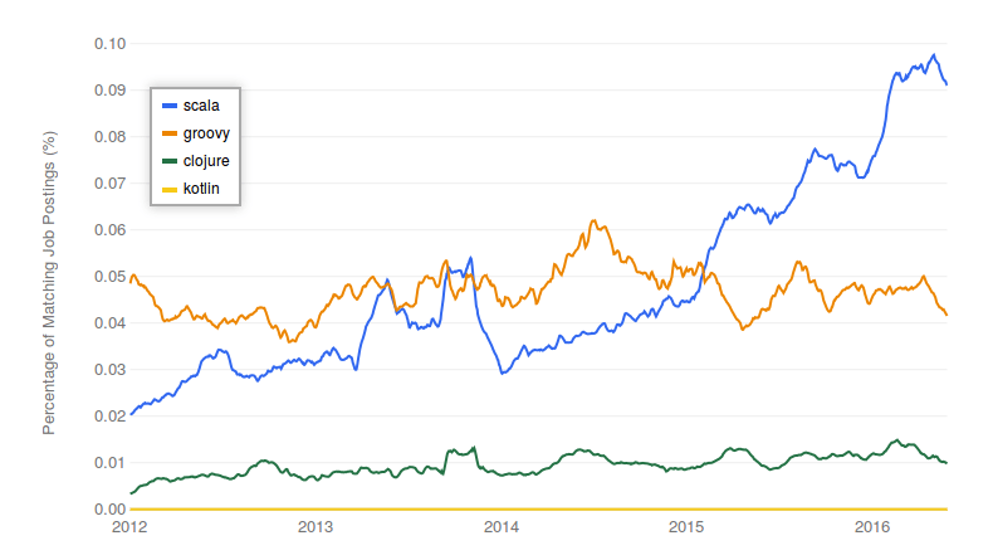The chart below describes the permanent jobs and Contract-based jobs available based on the knowledge of Scala Programming Language.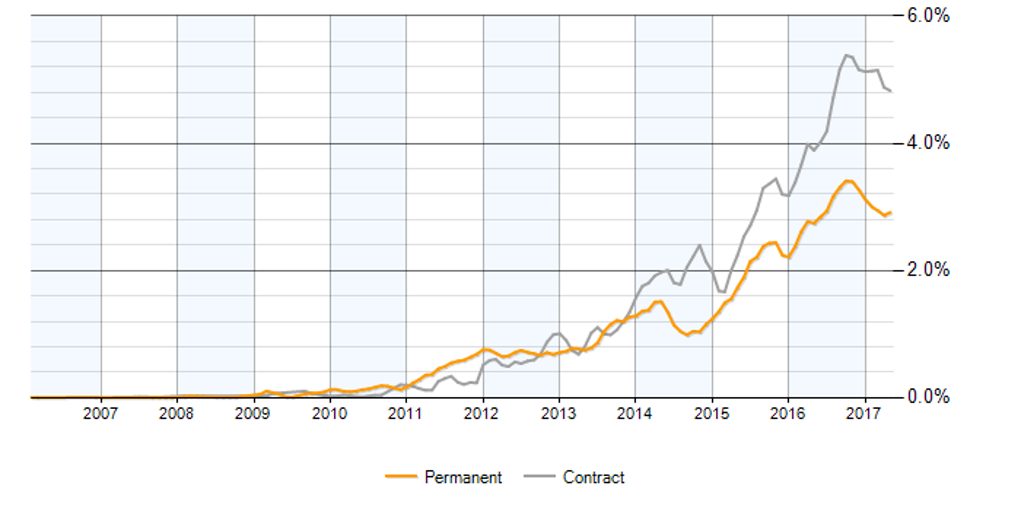So, with this, we come to an end of this What is Scala language.

I hope we sparked a little light upon your knowledge about Scala, its features and the various types of operations that can be performed using Scala.

#Last but not least, ask for help!

# Data Visualization With MatPlotLib

• MatPlotLib is one of the most important library, provided by python for data visualization.
• It supports both 2Dimentional and 3Dimensional graphics.
• It makes use for NumPy for mathematical operations.
• It facilitates an object-oriented API that helps in embedding plots in applications using Python GUI toolkits like PyQt, WxPythonotTkinter.
• Matplotlib requires a large set of dependencies:
• Python (>= 2.7 or >= 3.4)
• NumPy
• setuptools
• dateutil
• pyparsing
• libpng
• pytz
• FreeType
• cycler
• six
• matplotlib.pyplot is a library containing the collection of command style functions that enable Matplotlib to work like MATLAB.

Types of Plots:

 Function name Description Bar Used to make a bar plot Barh Used to create a Horizontal bar plot Boxplot Used to create box and whisker plot Hist Used to create a histogram Hist2d Used to create a 2D histogram Pie Used to create a Pie chart Polar Used to create a polar plot Scatter Used to create scatter plot of x vs y Stackplot Used to create a stacked area plot Stem Used to create a Stem plot Step Used to make a Step plot Quiver Used to plot a 2D field of arrows

• Image Functions:
 Function name Description Imread Used to read an image from a file into an array Imsave Used to save an array as in image file. Imshow Used to Display an image on the axes

• Axis Function:
 Axes Add axes to an figure Text Used to add an text to the axes Title Used to set a title to a current axes Xlable or Ylabel Used to set label to x- axis or y-axis X-lim or Y-lim Used to set limit to x-axis or y-axis Xticks or Yticks Used Get or set the x-limits or y-limit of the current tick locations and labels.

• Figure Functions:

 Function Name Description Figtext It adds text to a figure Figure Used to create new figure Show Used to display a figure Savefig Used to save current figure Close Used to close a figure

• Figure Class in MatPlotLib:

• The matplotlib.figure package contains the Figure class.
• It is a top-level container for all kinds of plot elements.
• The Figure object is instantiated by calling the function figure() from the pyplot package.
• Syntax: fig1=plt.figure()
• Parameters of the figure() are as follows:
 Name Description Figsize Displays width and height in inches Dpi Dots per inches Facecolor Figure patch facecolor Edgecolor Figure patch edge color Linewidth Edge line width

• Axes Class in MatplotLib:

• Axes object is a region of the image with the data space.
• A particular figure may contain many Axes, but a given Axes object can only be in a single Figure.
• The Axes contains two or three Axis objects respective to its dimensions. The Axes class and its member functions are the basic entry point to working with the Object-Oriented interface.
• Axes object is added to the figure by calling a function add_axes().
• It returns the axes object and adds an axes at position rect [left, bottom, width, height] where all parameters are in fractions of figure width and height.
• legend(): Used to label the data with a specific name.
• syntax : ax.legend(handles, labels, loc)
• Where labels are a sequence of strings and handle the sequence of Line2D or Patch instances. loc can be a string or an integer denoting the legend location.
 Location String Location code Best 0 Upper right 1 Upper left 2 Lower right 3 Lower left 4 Right 5 Center-left 6 Center-right 7 Lower center 8 Upper center 9 Center 10

• axes.plot(): It plots values of an array vs another as lines or markers.

• The plot() method can have an optional format string argument to specify a specific color, style, and size of line and marker.

 Character Color ‘b’ Blue ‘g’ Green ‘r’ Red ‘k’ Black ‘c’ Cyan ‘m’ Magenta ‘y’ Yellow ‘w’ White

• Marker codes:
 Character Description ‘.’ Point marker ‘o’ Circle marker ‘x’ x marker ‘D’ Diameter marker ‘H’ Hexagon marker ‘s’ Square marker ‘+’ Plus marker

• Line Styles:
 Character Description ‘-’ Solid line ‘—’ Dashed line ‘-.’ Dash-dot line ‘:’ Dotted line

Ex) import matplotlib.pyplot as plt

y = [1, 5, 10, 17, 25,36,49, 64]

x1 = [1, 16, 30, 42,55, 68, 77,88]

x2 = [1,6,12,18,28, 40, 52, 65]

fig = plt.figure()

ax = fig.add_axes([0,0,1,1])

l1 = ax.plot(x1,y,’rs-‘) # solid line with yellow colour and square marker

l2 = ax.plot(x2,y,’mo–‘) # dash line with green colour and circle marker

ax.legend(labels = (‘hadoop’, ‘datascience’), loc = ‘lower right’) # legend placed at lower right

ax.set_title(“jobs as per sector”)

ax.set_xlabel(‘medium’)

ax.set_ylabel(‘sales’)

plt.show()

Output: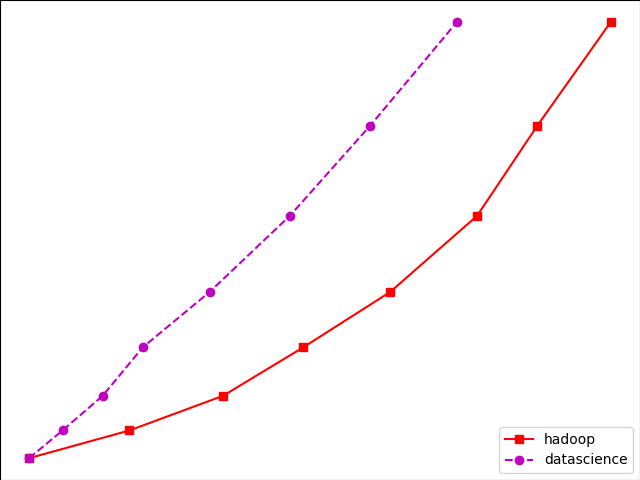• Multiplot: We can plot multiple graphs into a single canvas using a multiple subplots.
•  Subplot() function returns axes object at a given grid position.
• Syntax: plt.subplot(subplot(nrows, ncols, index)

Ex) import matplotlib.pyplot as plt

fig,a =  plt.subplots(2,2)

import numpy as np

x = np.arange(2,8)

a.plot(x,x**2)

a.set_title(‘square’)

a.plot(x,np.sqrt(x))

a.set_title(‘square root’)

a.plot(x,np.exp(x))

a.set_title(‘exp’)

a.plot(x,np.log10(x))

a.set_title(‘log’)

plt.show()

OutPut: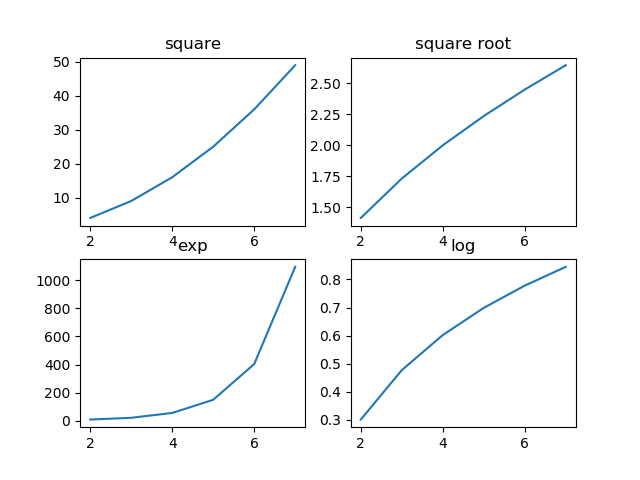• Subplot2grid() Function:

• It gives more flexibility in creating an axes object at a particular location of the grid.

• It also allows the axes object to be spanned across multiple rows or cols.
• Syntax:

Plt.subplot2grid(shape, location, rowspan, colspan)

Ex) import matplotlib.pyplot as plt

a1 = plt.subplot2grid((4,4),(0,0),colspan = 2)

a2 = plt.subplot2grid((4,4),(0,2), rowspan = 3)

a3 = plt.subplot2grid((4,4),(1,0),rowspan = 2, colspan = 2)

import matplotlib.pyplot as plt

a1 = plt.subplot2grid((4,4),(0,0),colspan = 2)

a2 = plt.subplot2grid((4,4),(0,2), rowspan = 3)

a3 = plt.subplot2grid((4,4),(1,0),rowspan = 2, colspan = 2)

import numpy as np

x = np.arange(1,10)

a2.plot(x, x**3)

a2.set_title(‘cube’)

a1.plot(x, np.exp(x))

a1.set_title(‘exp’)

a3.plot(x, np.log(x))

a3.set_title(‘log’)

plt.tight_layout()

plt.show()

import numpy as np

x = np.arange(1,10)

a2.plot(x, x**3)

a2.set_title(‘cube’)

a1.plot(x, np.exp(x))

a1.set_title(‘exp’)

a3.plot(x, np.log(x))

a3.set_title(‘log’)

plt.tight_layout()

plt.show()

OutPut: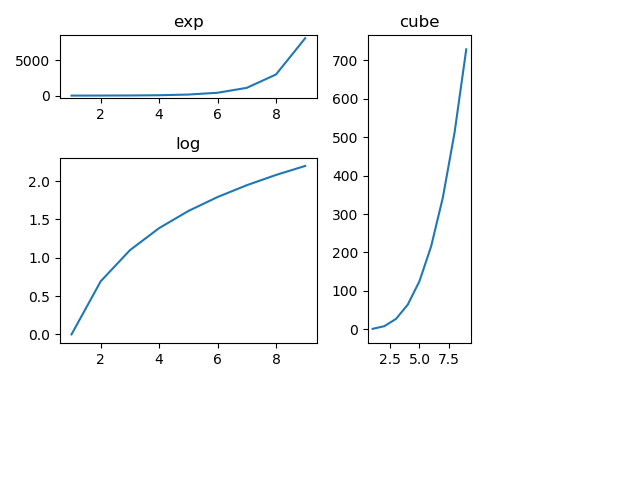• Grid():
• The grid() function of the axes object sets the visibility of the grid inside a figure to on or off. You can also display major/minor (or both) ticks of the grid.
• Additionally, color, line style, and linewidth properties can be set in the grid() function.

Ex) import matplotlib.pyplot as plt

import numpy as np

fig, axes = plt.subplots(1,3, figsize = (12,4))

x = np.arange(2,22)

axes.plot(x, x**4, ‘g’,lw=2)

axes.grid(True)

axes.set_title(‘default grid’)

axes.plot(x, np.exp(x), ‘r’)

axes.grid(color=’b’, ls = ‘-.’, lw = 0.25)

axes.set_title(‘custom grid’)

axes.plot(x,x)

axes.set_title(‘no grid’)

fig.tight_layout()

plt.show()

OutPut: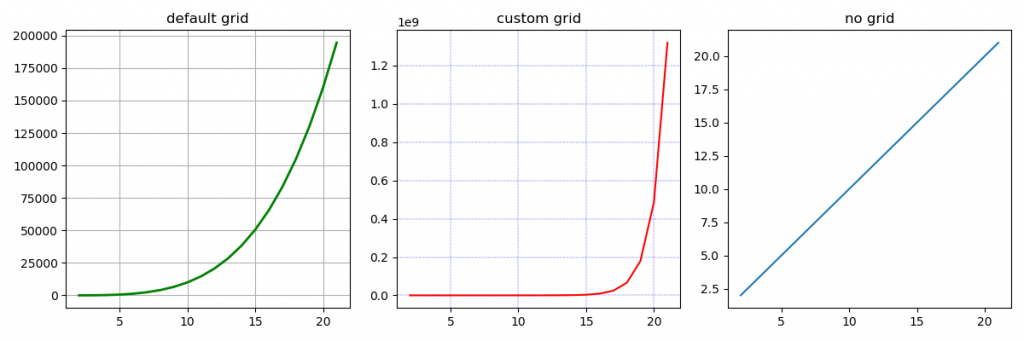• Setting Limits:

This function used to set x axis limit and y-axis limit in the graph.

Ex) import matplotlib.pyplot as plt

fig = plt.figure()

a1 = fig.add_axes([0,0,1,1])

import numpy as np

x = np.arange(1,90)

a1.plot(x, np.exp(x),’r’)

a1.set_title(‘exp’)

a1.set_ylim(0,80000)

a1.set_xlim(0,10)

plt.show()

Output: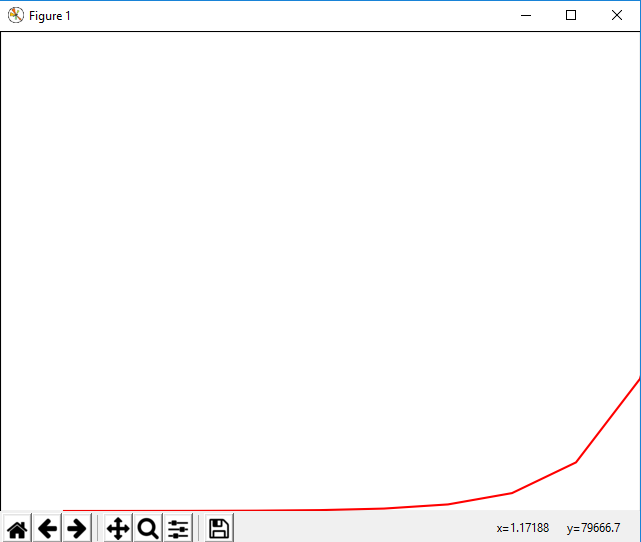• Setting Ticks and Tick Labels:

• This method will mark data points at the given positions with ticks.
• The xticks() and yticks() functions take the list of the object as an argument.
• Similarly, labels corresponding to  the tick marks can be set using set_xlabels() and set_ylabels() functions respectively.

Ex)

import matplotlib.pyplot as plt

import numpy as np

import math

x = np.arange(0, math.pi*4, 0.04)

fig = plt.figure()

ax = fig.add_axes([0.2, 0.4, 0.16, 0.26]) # main axes

y = np.sin(x)

ax.plot(x, y)

ax.set_xlabel(‘angle’)

ax.set_title(‘sine’)

ax.set_xticks([0,3,6,9])

ax.set_xticklabels([‘a’,’b’,’c’,’d’])

ax.set_yticks([-1,0,1])

plt.show()

Output: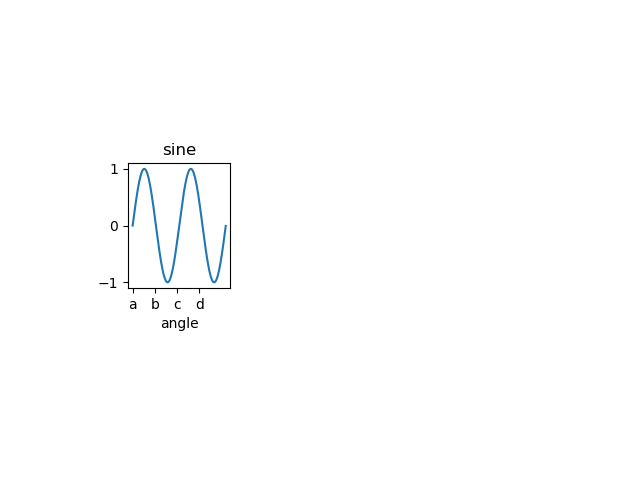• Bar Plot: Used to present the data using rectangular bars with height and width proportional to the value.
• It can be plotted vertically as well as horizontally.
• Syntax: ax.bar(x, height, width, bottom, align)

• The function makes a bar plot with a bound rectangle of size (x −width = 2; x + width=2; bottom; bottom + height).

• Parameters of bar() functions:

 Name Description x the sequence of scalars represents an x coordinates of the bars. align controls if x is the bar center (default) or left edge. height The sequence of scalars represents the height(s) of the bars. width The width(s) of the bars default 0.8 bottom y coordinate(s) of the bars default None. align {‘center’, ‘edge’}, optional, default ‘center’

Ex)

import numpy as np

import matplotlib.pyplot as plt

N = 5

boysMeans = (120, 135, 130, 135, 127)

girlsMeans = (125, 132, 134, 120, 125)

ind = np.arange(N) # the x locations for the groups

width = 0.35

fig = plt.figure()

ax = fig.add_axes([0,0,1,1])

ax.bar(ind, boysMeans, width, color=’r’)

ax.bar(ind, girlsMeans, width,bottom=boysMeans, color=’b’)

ax.set_ylabel(‘Scores’)

ax.set_title(‘Scores by group and gender’)

ax.set_xticks(ind, (‘G1’, ‘G2’, ‘G3’, ‘G4’, ‘G5’))

ax.set_yticks(np.arange(0, 81, 10))

ax.legend(labels=[‘boys’, ‘girls’])

plt.show()

OUTPUT: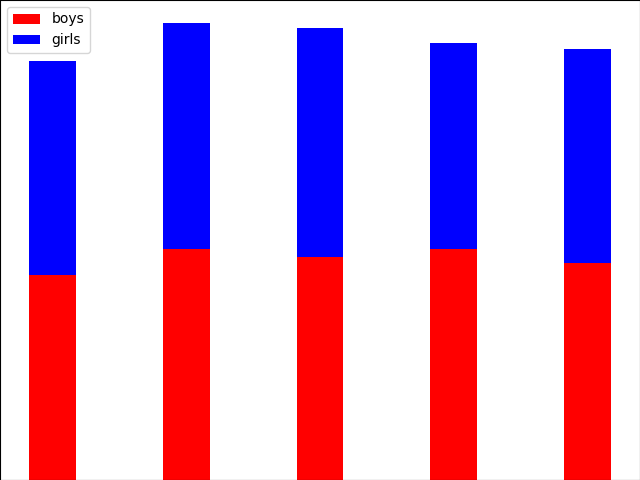• Histogram

• A histogram is an accurate representation of the distribution of a numerical data set.
• It is an estimation of the probability distribution of a continuous variable.
• Follow these steps to construct a histogram:
• Bin the range of values.
• Divide the entire range of values into a series of intervals.
• Count how many values fall into each interval.
•  Parameters:
 x array or a sequence of arrays bin integer or sequence range It specifies the lower and upper range of the bins density If True, then the first element of the return tuple will be counts normalized to form a probability density

Ex)

from matplotlib import pyplot as plt

import numpy as np

fig,ax = plt.subplots(1,1)

a = np.array([22,87,5,43,56,73,55,54,11,20,51,5,79,31,27])

ax.hist(a, bins = [0,25,50,75,100])

ax.set_title(“histogram of result”)

ax.set_xticks([0,25,50,75,100])

ax.set_xlabel(‘marks’)

ax.set_ylabel(‘no. of students’)

plt.show()

Output:

• Pie Chart:
• A Pie Chart can only display a series of data. Pie charts display the size of items in a data series, proportional to sum of its items.
• The data points in the pie chart are displayed as a percentage of the whole pie.
• Matplotlib API has a pie() function that creates a pie chart representing data in an array.
• The fractional area of each item in a data set is given by x/sum(x). If the sum(x)< 1, then the values of x display the fractional area directly and the array will not be normalized. The resulting pie will have an empty wedge of size 1 – sum(x).

• Parameters:
 Name Description x array-like label A sequence of strings providing labels for each wedge. Colors A sequence of matplotlib color arguments through which the pie chart will cycle. If None, then will use the colors in the currently active cycle. Autopct string used to label the items with their numeric value.  The label will be placed inside a wedge.  The format string will be fmt%pct.

Ex)

from matplotlib import pyplot as plt

import numpy as np

fig = plt.figure()

ax = fig.add_axes([0,0,1,1])

ax.axis(‘equal’)

langs = [‘Statistics’, ‘Python’, ‘Machine Learning’, ‘SQL’, ‘Big Data’]

students = [53,27,25,19,12]

ax.pie(students, labels = langs,autopct=’%1.2f%%’)

plt.show()

OutPut: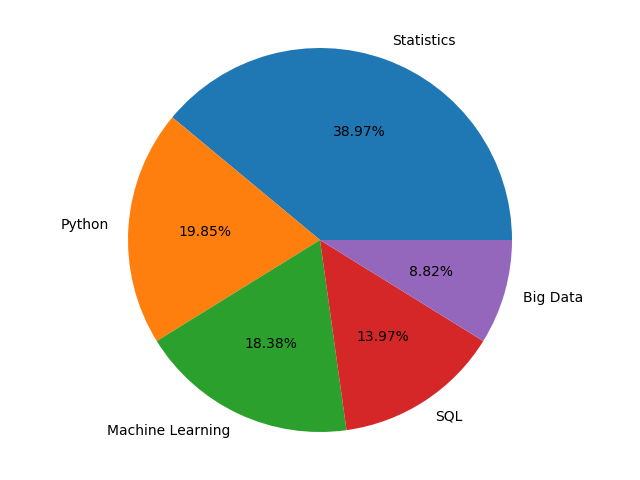• Scatter Plot:
• Scatter plots are used to plot data points over a horizontal and a vertical axis in an attempt to display how much one variable is affected by another.
• Each row in the data table is represented by a marker the position depends on its values in the columns set on the X and Y axes.
• A third variable may be set to correspond to color or size of markers, thus adding yet another dimension to the plot.

Ex)

import matplotlib.pyplot as plt

girls_marks = [19, 30, 10, 69, 80, 60, 14, 30, 80, 34]

boys_marks = [90, 29, 49, 48, 100, 48, 38, 45, 20, 30]

grades_range = [10, 20, 30, 40, 50, 60, 70, 80, 90, 100]

fig=plt.figure()

ax=fig.add_axes([0,0,1,1])

ax.scatter(grades_range, girls_marks, color=’r’)

ax.scatter(grades_range, boys_marks, color=’b’)

ax.set_xlabel(‘Grades Range’)

ax.set_ylabel(‘Grades Scored’)

ax.set_title(‘scatter plot’)

plt.show()

OutPut: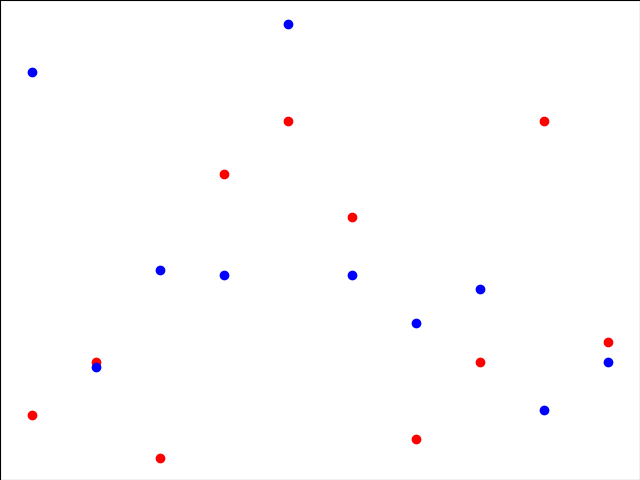• Contour Plot:
• Contour plots it is sometimes called as a Level Plots are a way to display a three-dimensional surface over a two-dimensional plane.
• It graphs two predictor variables X, Y on the y-axis and a response variable Z as contours. These contours sometimes are called as z-slices or an iso-response values.
• A contour plot is appropriate when you need to see how a value Z changes as a function of two inputs X and Y, i.e.  Z = f(X, Y).
•  A contour line or isoline of a function for two variables is a curve along which a function has a constant value.
• The independent variables x and y are usually restricted to a regular grid called meshgrid.
• The numpy.meshgrid creates a rectangular grid out of an array of x values and an array of y values.
• Matplotlib API also contains contour() and contourf() functions that draw a contour lines and filled contours, respectively.
• Both functions need 3 parameters x, y, and z.

EX)

import numpy as np

import matplotlib.pyplot as plt

xlist = np.linspace(-8.0, 10.0, 100)

ylist = np.linspace(-8.0, 10.0, 100)

X, Y = np.meshgrid(xlist, ylist)

Z = np.sqrt(X**4 + Y**4)

fig,ax=plt.subplots(1,1)

cp = ax.contourf(X, Y, Z)

fig.colorbar(cp) # Add a colorbar to a plot

ax.set_title(‘Filled Contours Plot’)

#ax.set_xlabel(‘x (cm)’)

ax.set_ylabel(‘y (cm)’)

plt.show()

OutPut: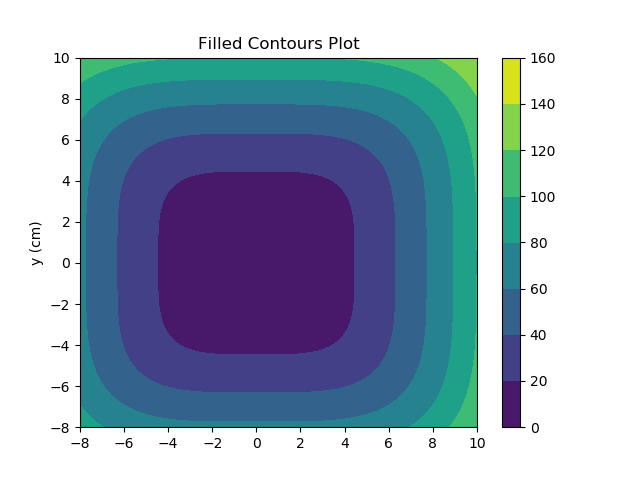• Quiver Plot:
• A quiver plot displays a velocity vectors as arrows with components as (u,v) at the points (x,y).
• quiver(x,y,u,v)
• Parameters:
 Name Description x The x coordinates of the arrow locations y The y coordinates of the arrow locations u The x components of the arrow vectors v The y components of the arrow vectors c The arrow colors

EX)

import matplotlib.pyplot as plt

import numpy as np

x,y = np.meshgrid(np.arange(-2, 2, .2), np.arange(-2, 2, .25))

z = x*np.exp(-x**2 – y**2)

v, u = np.gradient(z, .2, .2)

fig, ax = plt.subplots()

q = ax.quiver(x,y,u,v)

plt.show()

Output: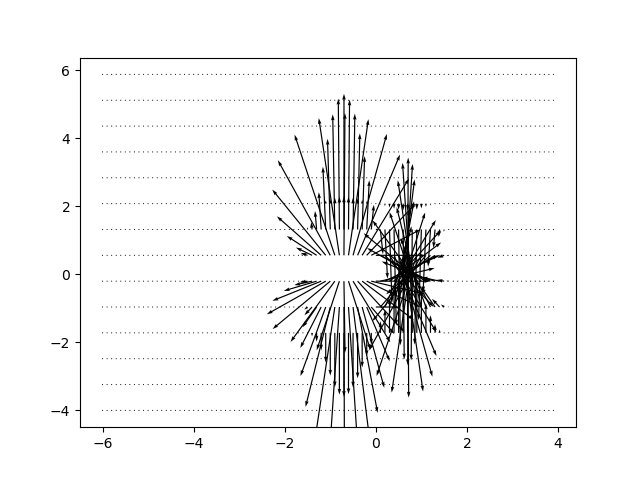• Box Plot:
• A box plot that is also called as a whisker plot displays a summary of a set of data containing the minimum, first quartile, median, third quartile, and maximum.
• In a box plot, we plat a box from the first quartile to the third quartile.
• A vertical line goes through the box from the median. The whiskers go from each quartile to the minimum or a maximum.

Ex)

import matplotlib.pyplot as plt

import numpy as np

value1 = [82, 76, 24, 40, 67, 62, 71, 79, 81, 22, 98, 89, 78, 67, 72, 82, 87, 66, 56, 52]

value2 = [12, 25, 11, 35, 36, 32, 96, 95, 3, 90, 95, 32, 27, 55, 100, 12, 1, 451, 37, 21]

value3 = [23, 89, 12, 78, 72, 89, 25, 69, 68, 86, 19, 49, 15, 16, 16, 75, 65, 31, 25, 52]

value4 = [99, 33, 75, 66, 83, 61, 82, 98, 10, 87, 29, 72, 26, 23, 72, 88, 78, 99, 75, 30]

box_plot_data = [value1, value2, value3, value4]

plt.boxplot(box_plot_data)

plt.show()

OutPut: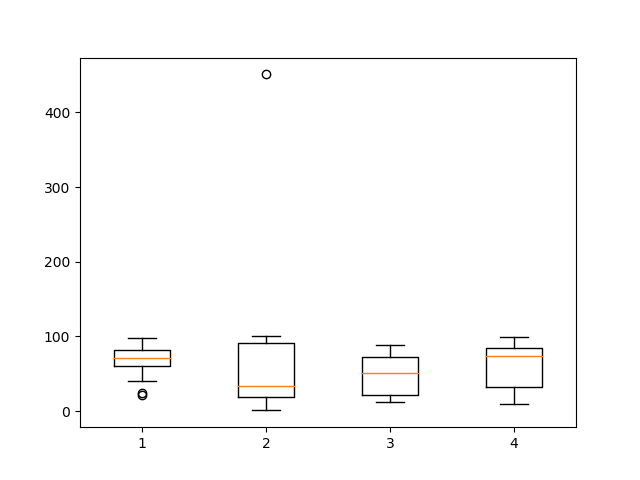• Violin Plot:
• Violin plots are just like box plots, except that they also display probability density of data at different values.
• These plots consist of a marker for the median of the data and a box indicating the interquartile range, similar to standard box plots.
• Overlaid over this box plot is a kernel density estimation.
• Like box plots, violin plots are used to display a comparison of a variable distribution or sample distribution across different categories.
• A violin plot is actually more informative than a plain box plot.
• In fact, while a box plot only shows summary statistics just like mean/median and interquartile ranges, whereas the violin plot shows the full distribution of the data.

EX)

import matplotlib.pyplot as plt

import numpy as np

np.random.seed(10)

collectn_1 = np.random.normal(300, 30, 300)

collectn_2 = np.random.normal(60, 10, 300)

collectn_3 = np.random.normal(60, 40, 300)

collectn_4 = np.random.normal(20, 25, 300)

# combine these different collections into a list

data_to_plot = [collectn_1, collectn_2, collectn_3, collectn_4]

# Create a figure instance

fig = plt.figure()

# Create an axes instance

ax = fig.add_axes([0,0,1,1])

# Create the boxplot

bp = ax.violinplot(data_to_plot)

plt.show()

OutPut: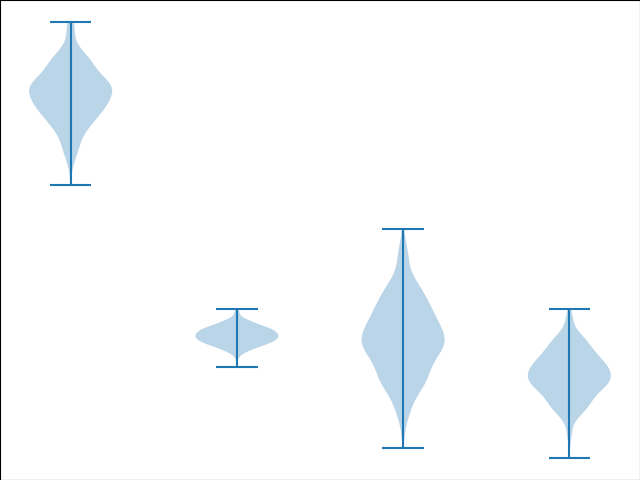Three Dimensional Plotting:

• All though Matplotlib was initially designed with only 2D plotting into consideration, still some 3D plotting utilities were built on top of Matplotlib’s 2D display in its later versions, to provide a set of tools for3D data visualization.
• 3D plots are enabled by importing the mplot3d toolkit, included with the Matplotlib package.

Ex)

from mpl_toolkits import mplot3d

import numpy as np

import matplotlib.pyplot as plt

fig = plt.figure()

ax = plt.axes(projection=’3d’)

z = np.linspace(0, 2, 200)

x = z * np.sin(40 * z)

y = z * np.cos(40 * z)

ax.plot3D(x, y, z, ‘red’)

ax.set_title(‘3D line plot’)

plt.show()

OutPut: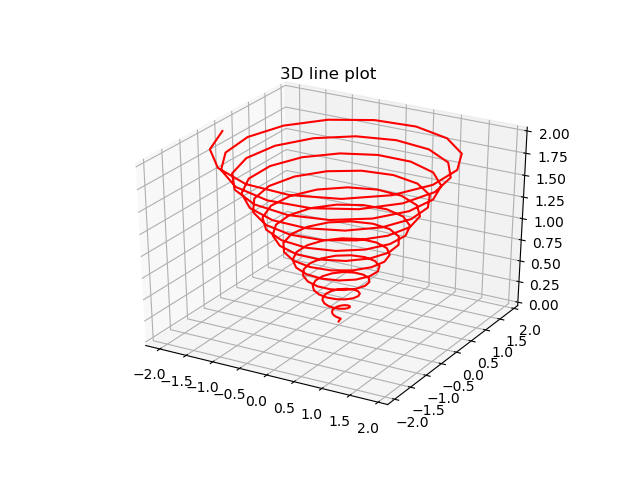• 3D Contour Plot:
• The ax.contour3D() function generates 3D contour plot.
• It needs all the input data to be in the form of two-dimensional regular grids, with the Z-data evaluated at each point.
• Here, we will display a 3D contour diagram of a 3D sinusoidal function.

EX)

from mpl_toolkits import mplot3d

import numpy as np

import matplotlib.pyplot as plt

def f(x, y):

return np.sin(np.sqrt(x ** 2 + y ** 2))

x = np.linspace(-4, 4, 40)

y = np.linspace(-4, 4, 40)

X, Y = np.meshgrid(x, y)

Z = f(X, Y)

fig = plt.figure()

ax = plt.axes(projection=’3d’)

ax.contour3D(X, Y, Z, 50, cmap=’binary’)

ax.set_xlabel(‘x’)

ax.set_ylabel(‘y’)

ax.set_zlabel(‘z’)

ax.set_title(‘3D contour’)

OutPut: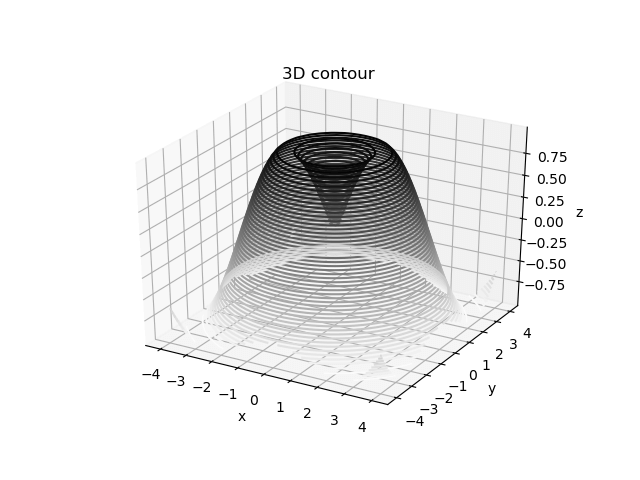• 3D Wireframe plot
• Wireframe plot takes a grid of elements and place it over the specified three-dimensional surface, and can make the resulting 3D forms quite easy to visualize.
• The plot_wireframe() function is used for this purpose

Ex)

from mpl_toolkits import mplot3d

import numpy as np

import matplotlib.pyplot as plt

def f(x, y):

return np.sin(np.sqrt(x ** 2 + y ** 2))

x = np.linspace(-4, 4, 40)

y = np.linspace(-4, 4, 40)

X, Y = np.meshgrid(x, y)

Z = f(X, Y)

fig = plt.figure()

ax = plt.axes(projection=’3d’)

ax.plot_wireframe(X, Y, Z, color=’green’)

ax.set_title(‘wireframe’)

plt.show()

plt.show()

OutPut: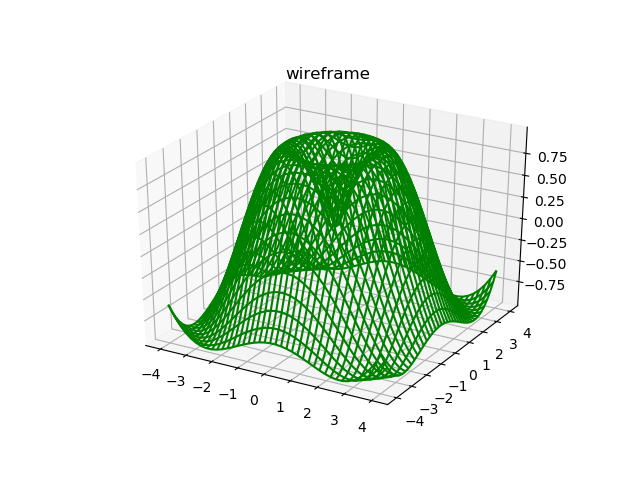• 3D Surface plot:
• Surface plot displays a functional relationship between a designated dependent variable (Y), and two independent variables (X and Z).
• The plot is a companion plot to the contour plot.
•  A surface plot is just like a wireframe plot, but each face of the wireframe is a filled polygon.
• The plot_surface() function x,y and z as arguments.

Ex)

from mpl_toolkits import mplot3d

import numpy as np

import matplotlib.pyplot as plt

x = np.outer(np.linspace(-4, 4, 40), np.ones(40))

y = x.copy().T # transpose

z = np.cos(x ** 2 + y ** 2)

fig = plt.figure()

ax = plt.axes(projection=’3d’)

ax.plot_surface(x, y, z,cmap=’viridis’, edgecolor=’none’)

ax.set_title(‘Surface plot’)

plt.show()

Output: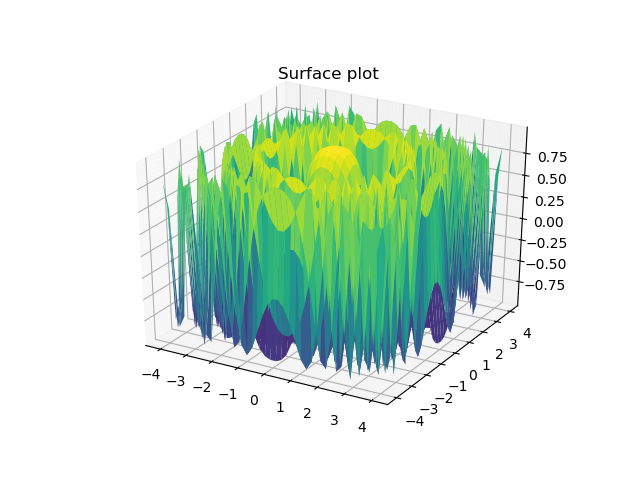## Amazon Elastic MapReduce (EMR)

### Amazon EMR: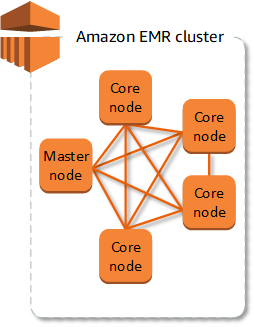► Amazon Elastic MapReduce (EMR) is an Amazon Web Services (AWS) tool for Big Data processing and analysis by using a cluster. Amazon EMR provides the expandable low-configuration service as an easier alternative to running in-house cluster computing.

Amazon EMR is a managed cluster platform that simplifies running big data frameworks, such as Apache and Apache Spark, on AWS to process and analyze vast amounts of data. By using these type of frameworks and related open-source projects, such as Apache Hive and Apache Pig, you can process the data for analytics purposes and business intelligence workloads regarding Big Data. Additionally, you can use Amazon EMR to transform and move large amounts of data means ‘Big Data’ into and out of other AWS data stores and databases, such as Amazon Simple Storage Service (Amazon S3) used for storage and retrieve the data and Amazon DynamoDB it’s is a fully managed NoSQL database service that works on key-value pair and other data structure documents provided by Amazon.

If you are not familiar with Amazon EMR, we recommend that you begin by reading the following, in addition to this section:

• Amazon EMR: This service page provides the Amazon EMR highlights, product details, and pricing information.
• Getting Started: Analyzing Big Data with Amazon EMR: These articles get you started using Amazon EMR quickly.

## Pandas

What is pandas?

• Pandas is an open-source Python Library That provides high-performance data manipulation and analyzing tool using its powerful data structures.
• The name Pandas comes from the word Panel Data – an Econometrics from Multidimensional data.
• Pandas library is built on over Numpy, that means Pandas needs Numpy to operate.
• Pandas provide an easy way to create, manipulate and wrangle the data.
• Pandas Helps us to perform following operations:
• Loading the Data
• Preparing the Data
• Manipulating the Data
• Modeling the Data
• Analyzing the Data
• Python with Pandas is used in different fields including academic and commercial domains that includes finance, economics, Statistics, analytics, etc.
• Features of Pandas:
• Fast and efficient DataFrame objects having default and customizable

indexing.

• Tools for loading data into the in-memory data objects from different file formats.
• Data alignment and integrated handling of missing data values.
• Reshaping and pivoting the date sets.
• Label-based on slicing, indexing and sub-setting of large data sets.
• Columns from data-structures can be inserted and deleted.
• Performing operations like groupBy over the dataset.

Installation of Pandas:

• For Mac OS:

Step1)         Open the terminal

Step2)         pip install pandas

• For Windows user:

Step1) Go to File menu

Step2) Go to settings

Step3) Go to Project

Step4) Go to project Interpreter

Step5) Click on ‘+’ icon

Step6) Type pandas.

Step7) Select it and install it.

Step8) import pandas as n

Step9) Use it

• Data Structures in Pandas:
• Series
• Data-Frames
• Panel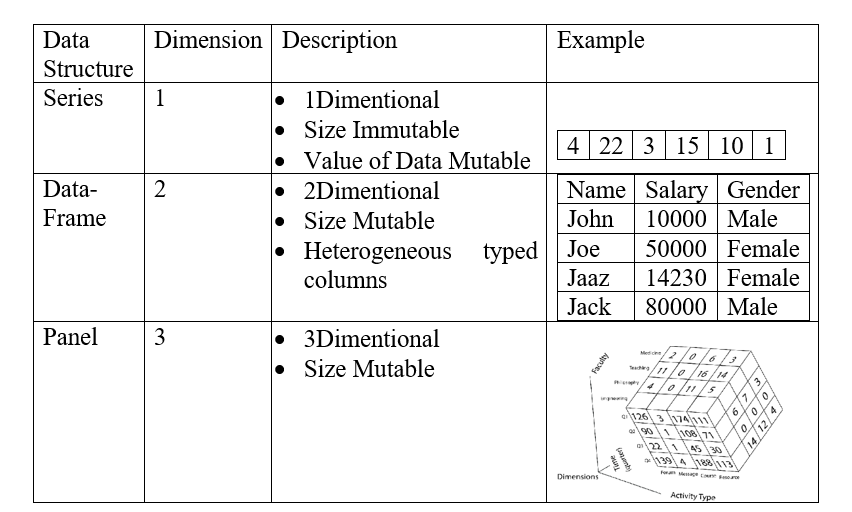• Mutability: All Pandas data structures are value mutable

and except Series all are also size mutable. Series is a size immutable.

• Series: Series is 1 Dimensional labelled array having the size Immutable and

Value of Data Mutable.

Syntax) pandas.Series(data,index,dtype,copy)

• data: it takes various forms like nD-array, list, constants
• index: Index value must be unique
• dtype: It is for datatype
• copy: It is used to copy the data. By default, its value is false.
• Series can be created using various inputs:
• Array:

If data is from an ndarray, then the index passed must be of the same length.

If no index is passed, then by default index will be range(n) where n is array length starting from zero,

i.e. [0,1,2,3…. range(len(array))-1].

Ex)    import pandas as pad

import numpy as num

a=num.array([1,4,5,6,7])

s=pad.Series(a)

print s

• Dictionary:

A dictionary can be passed as an input and if no index is specified, then the dictionary keys are taken in a sorted order to construct its index. If index is passed, the values in data corresponding to the labels present in the index will be pulled out.

Ex)    import pandas as pad

import numpy as num

a= {‘a’: ‘add’, ‘s’: ‘sub’, ‘d’: ‘dvd’}

s=pad.Series(a)

print s

• Constants:

If data is a constant, then an index must be provided. The value will be repeated to match the length of index.

Ex)    import pandas as pad

import numpy as num

s=pad.Series(4,index=[0,1,2])

print s

Accessing Data from Series with Position:

Ex)    import pandas as pad

import numpy as num

a=num.array([1,4,5,6,7])

s=pad.Series(a)

print s

• DataFrames: It is a 2Dimentional array which is Size Mutable and Heterogeneous typed columns.
• Syntax:pandas.DataFrame(data, index, column, dtype, copy)
• Data: it takes values in various forms like array, series, map, list, dictionary, constants and also another DataFrame.
• index: For the row labels, the Index is used for the resulting frame, it is Optional Default np.arrange(n) if no index is passed.
• Column: In column labels, the optional default syntax is – np.arrange(n). It is only true if no index is passed.
• Dtype: It denotes datatype of each column.
• Copy: It is used for copying of data, by default it is false.
• DataFrames can be created using various inputs:
• List:

Ex)import pandas as pad

data = [9,2,3,4,5]

df1 = pad.DataFrame(data)

print df

• Dictionary:

Ex)    import pandas as pad

import numpy as num

a= {‘a’: ‘add’, ‘s’: ‘sub’, ‘d’: ‘dvd’}

df=pad.DataFrame(a)

print df

• Series:

Ex)    import pandas as pad

import numpy as num

a= {[‘a’, ‘add’], [‘s’, ‘sub’], [‘d’, ‘dvd’]}

df=pad.Series(‘sr’, ‘opp’)

print df

• Numpy ndimetional array:

Ex)    import pandas as pad

import numpy as num

a= [1,2,3,4,5]

s=pad.DataFrame(a)

print df

• Another DataFrame:

Ex)    import pandas as pad

import numpy as num

a= {‘a’: ‘add’, ‘s’: ‘sub’, ‘d’: ‘dvd’}

s=pad.DataFrame(a)

print s

• Column additions:

Ex) import pandas as pad

d = {‘one’ : pad.Series([2, 3, 4], index=[‘a’, ‘b’, ‘c’]),

‘two’ : pad.Series([2, 3, 4, 5], index=[‘a’, ‘b’, ‘c’, ‘d’])}

df = pad.DataFrame(d)

print (“Adding a new column by passing as Series:”)

df[‘three’]=pad.Series([100,200,300],index=[‘a’,’b’,’c’])

print df

print (“Adding a new column using the existing columns in DataFrame:”)

df[‘four’]=df[‘one’]+df[‘three’]

print df

• Column Deletion: It can be done using either del() or pop().

Ex) import pandas as pd

d = {‘one’ : pd.Series([2, 3, 4], index=[‘a’, ‘b’, ‘c’]),

‘two’ : pd.Series([2, 3, 4, 5], index=[‘a’, ‘b’, ‘c’, ‘d’]),

‘three’ : pd.Series([100,200,300], index=[‘a’,’b’,’c’])}

df = pd.DataFrame(d)

print (“Our dataframe is:”)

print df

# using del function

print (“Deleting the first column using DEL function:”)

del df[‘one’]

print df

# using pop function

print (“Deleting another column using POP function:”)

df.pop(‘two’)

print df

Panel:

• A panel is a 3D container of data elements. The term Panel data is been derived from econometrics and is partially responsible for the name pandas − pan(el)-da(ta)-s.
• The names for the 3 axes are deliberated to give some semantic meaning to describe operations involving a panel data.
• Items: axis zero, each item corresponds to a DataFrame contained inside.
• major_axis: axis one, it is the index (rows) of each of the DataFrames.
• minor_axis: axis two, it is the columns of each of the DataFrames.
• Syntax) pandas.Panel(data, items, major_axis, minor_axis, dtype, copy)
• Data: Data can taken from various forms like array, series, map, lists, dictionary, constants and also another DataFrame.
• Items: axis zero, each item corresponds to a DataFrame contained inside.
• major_axis: axis one, it is the index (rows) of each of the DataFrames.
• minor_axis: axis two, it is the columns of each of the DataFrames.
• dtype: It describes the datatype of each column.
• copy: copy the data. By default, its value is false.

## ·       Create Panel

A Panel can be created using multiple ways like:

o   From ndimentional array

Ex)    import pandas as pad

import numpy as num

data = num.random.rand(6,8,1)

p = pd.Panel(data)

print p

o   From dictionary of DataFrame

Ex)    import pandas as pd

import numpy as np

data = {‘Item1’ : pd.DataFrame(np.random.randn(4, 3)),

‘Item2’ : pd.DataFrame(np.random.randn(4, 2))}

p = pd.Panel(data)

print p

·         Selecting the Data from Panel

o   Using Items:

Ex)     import pandas as pad

import numpy as np

data = {‘Item1’ : pad.DataFrame(np.random.randn(5, 3)),

‘Item2’ : pd.DataFrame(np.random.randn(5, 2))}

p = pd.Panel(data)

print p[‘Item1’]

o   Using major_axis:

Ex)      import pandas as pd

import numpy as np

data = {‘Item1’ : pd.DataFrame(np.random.randn(9, 3)),

‘Item2’ : pd.DataFrame(np.random.randn(9, 2))}

p = pd.Panel(data)

print p.major_xs(1)

• Using minor_axis:

Ex)     import pandas as pd

import numpy as np

data = {‘Item1’ : pd.DataFrame(np.random.randn(8, 3)),

‘Item2’ : pd.DataFrame(np.random.randn(8, 2))}

p = pd.Panel(data)

print p.minor_xs(1)

• Series: Basic Funtions:
 Name Description Example Axes Used to return the list of the labels of the series. s = pd.Series(np.random.randn(9)) print s.axes Empty It returns the Boolean value about whether the Object is empty or not. True will indicate that the object is empty. s = pd.Series(np.random.randn(9)) print s.empty Ndim It returns the number of dimensions of the object. s = pd.Series(np.random.randn(9)) print s.ndim Size It returns length of series s = pd.Series(np.random.randn(9)) print s.size Values It returns the actual data present in series s = pd.Series(np.random.randn(9)) print s.values head() It returns first n records from the series s = pd.Series(np.random.randn(9)) print s.head(3) tail() It returns last n records from series s = pd.Series(np.random.randn(9)) print s.tail(3)

• Basic DataFrame Functions:
 Name Description Example T Transposes rows and column df = {‘Name’:[‘Tom’, ‘dick’, ‘harry’ ], ‘Age’:[20, 21, 19]} print df.T Axes It returns list rows and column label axis df = {‘Name’:[‘Tom’, ‘dick’, ‘harry’ ], ‘Age’:[20, 21, 19]} print df.axis Dtypes Returns data type of each column. df = {‘Name’:[‘Tom’, ‘dick’, ‘harry’ ], ‘Age’:[20, 21, 19]} print df.dtypes Empty Returns whether the DataFrame is empty using Boolean value df = {‘Name’:[‘Tom’, ‘dick’, ‘harry’ ], ‘Age’:[20, 21, 19]} print df.empty Ndim Returns number of dimensions i.e. 2D df = {‘Name’:[‘Tom’, ‘dick’, ‘harry’ ], ‘Age’:[20, 21, 19]} print df.ndim Shape Returns a tuple representing dimensionality of the DataFrame. df = {‘Name’:[‘Tom’, ‘dick’, ‘harry’ ], ‘Age’:[20, 21, 19]} print df.shape Size Returns the number of elements present df = {‘Name’:[‘Tom’, ‘dick’, ‘harry’ ], ‘Age’:[20, 21, 19]} print df.size Values Returns actual data df = {‘Name’:[‘Tom’, ‘dick’, ‘harry’ ], ‘Age’:[20, 21, 19]} print df.values Head Returns the top n records df = {‘Name’:[‘Tom’, ‘dick’, ‘harry’ ], ‘Age’:[20, 21, 19]} print df.head(2) tail Return the bottom n records df = {‘Name’:[‘Tom’, ‘dick’, ‘harry’ ], ‘Age’:[20, 21, 19]} print df.tail(2)

• Pandas – Descriptive Statistics

Ex)    import pandas as pd

import numpy as np

#Create a Dictionary of series

d1 = {‘Name’:pd.Series([‘Tomy’,’Jimy’,’Ricky’,’Viny’,’Steven’,’Smithen’,’Jacky’,

‘Lee’,’Dravid’,’Gaspery’,’Betin’,’Andru’]),

‘Age’:pd.Series([22,23,26,21,30,29,23,34,40,30,51,46]),    ‘Rating’:pd.Series([5.23,3.44,3.95,2.66,4.20,4.6,6.8,1.78,3.98,4.80,4.10,3.65])

}

#Create a DataFrame

df = pd.DataFrame(d)

 Name Description Example count() Counts number of not null observations print df.count() sum() Sums the values print df.sum() mean() Finds mean of the values print df.mean() median() Find medians of the values print df.median() mode() Finds modes of the values print df.mode() std() Finds standard deviation of the values print df.std() min() Finds minimum value from given data print df.min() max() Finds maximum value from given data print df.max() abs() Finds absolute value print df.abs() prod() Gives product of the values print df.prod() cumsum() Gives the cumulative summation print df.cumsum() cumprod() Gives the cumulative product print df.cumprod()
• Iterations in Pandas:

The behavior of basic iteration over Pandas objects depends on its type. i.e. when iterating over a Series, it is regarded as array-like, and basic iteration displays the values.

In other data structures, like DataFrame and Panel, follow the dictionary like convention of iterating over the keys of  objects.

• Iteration functions over DataFrames:

import pandas as pd

import numpy as np

N=20

df = pd.DataFrame({

‘D’: pd.date_range(start=’2019-08-01′,periods=N, frequency=’D’),

‘z’: np.linspace(0,stop=N-1,num=N),

‘c’: np.random.rand(N),

‘W’: np.random.choice([‘Low’, ‘Medium’, ‘High’],N).tolist(),

‘R’: np.random.normal(900, 90, size=(N)).tolist()

})

 Name Description Example iteritems() Used to iterate over the (key,value) pairs for key,value in df.iteritems(): print key,value iterrow() It returns iterator yielding each index value along with a series containing the data in each row. for row_index,row in df.iterrows(): print row_index,row itertuples() It returns an iterator yielding a named tuple for each row in a DataFrame. for row in df.itertuples(): print row

Pandas methods to work with textual data:

Ex) import pandas as pd

import numpy as np

s = pd.Series([‘Tom’, ‘Dick’, ‘Harry’, ‘Allen’, np.nan, ‘6234’,’SteveJobs’])

 Name Description Example lower() Converts all characters into lower case print s.str.lower() upper() Converts all characters into upper case print s.str.upper() len() Displays total number of characters present in a string print s.str.len() strip() Helps to strip whitespace(including newline) from each string in the Series from both the sides. print s.str.strip() split(‘ ’) Splits each string according to given delimiter print s.str.split(‘ ’) cat(sep=‘ ’) Concatenates the series elements with given separator. print s.str.cat(sep=‘’) get_dummies() It returns the Data-Frame with One-Hot Encoded values. print s.str.get_dummies() contains(pattern) Returns true if given pattern is present print s.str.contains() replace(a,b) Replaces the value of a with b print s.str.replace(‘@’,’\$’) repeat(value) Repeats each element for the specific number of times print s.str.repeat(2) count(pattern) Returns the count of particular element present print s.str.count(‘s’) startswith(pattern) Returns true if string starts with the given pattern print s.str.startswith(‘I’) endswith(pattern) Returns true if string ends with the given pattern print s.str.endswith(‘m’) find(pattern) Returns first position of first occurrence print s.str.find(‘r’) findall(pattern) Returns all occurrence of a substring print s.str.findall(‘ra’) swapcase Swaps from lower to upper case or viz versa print s.str.swapcase() islower() Returns true if all characters are in lower case print s.str.islower() isupper() Returns true if all characters are in upper case print s.str.upper() isnumeric() Returns true if all characters are numeric print s.str.numeric()

• Pandas – Window Statistics Functions:

For working over numerical data, Pandas provide some variants like rolling, expanding and exponentially moving weights for window statistics. Among these are some like sum, mean, median, variance, covariance, correlation, etc.

• .rolling() Function: This function can be applied on series of data. Specify window=n argument and apply appropriate statistical function on top of it.

Ex)       import pandas as pd

import numpy as np

df = pd.DataFrame(np.random.randn(10, 4),

index = pd.date_range(‘2/2/2022’, periods=5),

columns = [‘W’, ‘X’, ‘Y’, ‘Z’])

print df.rolling(window=4).mean()

Output)               A                     B                       C                D

2022-02-01        NaN               NaN                NaN         NaN

2022-02-02        NaN                NaN                 NaN         NaN

2022-02-03          NaN              NaN                  NaN         NaN

2022-02-04   0.628267   -0.047040   -0.287467   -0.161110

2022-02-05   0.398233    0.003517    0.099126   -0.405565

Since the window size is 4, for first three elements there are nulls and from fourth the value will be the average of the n, n-1 and n-2 elements.

• .expanding() Function:

This function can be applied on series of data. Specify the min_periods=n arguments and apply appropriate statistical function on top of it.

Ex)       import pandas as pd

import numpy as np

df = pd.DataFrame(np.random.randn(10, 4),

index = pd.date_range(‘2/2/2022’, periods=5),

columns = [‘W’, ‘X’, ‘Y’, ‘Z’])

print df.expanding(min_periods=3).mean()

Output)                     A                 B             C           D

2022-02-01        NaN         NaN         NaN         NaN

2022-02-02        NaN         NaN         NaN         NaN

2022-02-03         NaN         NaN         NaN         NaN

2022-02-04   0.628267   -0.047040   -0.287467   -0.161110

2022-02-05   0.398233    0.003517    0.099126   -0.40556

• .ewm() Function: ewm is applied over a series of data. Specify any of com, span, halflife argument and apply appropriate statistical function on top of it. It assigns the weights exponentially.

Ex)    import pandas as pd

import numpy as np

df = pd.DataFrame(np.random.randn(10, 4),

index = pd.date_range(‘2/2/2022’, periods=5),

columns = [‘W’, ‘X’, ‘Y’, ‘Z’])

print df.ewm(com=0.5).mean()

OutPu t                       A               B               C                  D

2022-02-01   1.088512   -0.650942   -2.547450   -0.566858

2022-02-02   0.865131   -0.453626   -1.137961    0.058747

2022-02-03  -0.132245   -0.807671   -0.308308   -1.491002

2022-02-04   1.084036    0.555444   -0.272119    0.480111

2022-02-05   0.425682    0.025511    0.239162   -0.153290

Window functions are majorly used while determining the trends within the data graphically by smoothing the curve. If there is a lot of variation in  everyday data and  lots of data points are available, then taking the samples and plotting is one approach and applying the window computations and plotting the graph on the results is another approach. By these methods, we can smooth the curve or the trend.

• Using SQL in Pandas:

import pandas as pd

url = ‘https://raw.github.com/pandasdev/

pandas/master/pandas/tests/data/tips.csv’

tips=pd.read_csv(url)

print tips.head()

 Condition Description Example Select With Pandas, column selection is done by passing a list of column names to your Data-Frame print tips[[‘total_bill’, ‘tip’, ‘smoker’, ‘time’]].head(5) Where Data-Frames can be filtered in multiple ways just like where condition in sql. print tips[tips[‘time’] == ‘Dinner’].head(5) GroupBy This operation fetches the count of records in each group throughout a dataset. print tips.groupby(‘sex’).size() Top N rows Returns top n records print tips.head(5)

• Performing Sql join in Pandas:
• Pandas provides a single function ‘merge()’, as the entry point for all standard database join operations between Data-Frame objects.

Ex)    import pandas as pd

left = pd.DataFrame({‘id’:[1,2,3,4,5], ‘Name’: [‘Ali’, ‘Any’,

‘Amen’, ‘Arik’, ‘Amy’],

‘subject_id’:[‘sub1′,’sub2′,’sub4′,’sub6′,’sub5’]})

right = pd.DataFrame({‘id’:[1,2,3,4,5],’Name’: [‘Bil’, ‘Briany’,

‘Bany’, ‘Brycy’, ‘Betten’],

‘subject_id’:[‘sub2′,’sub4′,’sub3′,’sub6′,’sub5’]})

 Name Description Example left join Displays common elements and elements of 1st dataframe print pd.merge(left, right, on=’subject_id’, how=’left’) right join Displays common elements and elements of 2nd dataframe print pd.merge(left, right, on=’subject_id’, how=’right’) outer join Displays entire elements of 1st  and 2nd dataframes print pd.merge(left, right, how=’outer’, on=’subject_id’) inner join Displays only common elements of 1st  and 2nd dataframes print pd.merge(left, right, on=’subject_id’, how=’inner’)

## NumPy

### ♦What is NumPy?

• NumPy is a array-processing package. It provides multidimensional array object, and tools for working with these arrays with high-performance.
• It contains various features as follows:
• A powerful N-dimensional array object
• Sophisticated (broadcasting) functions
• Tools for integrating C/C++ and Fortran code

Useful linear algebra, Fourier transform, and random number capabilities

• NumPy can also be used as an efficient multi-dimensional container of generic data.
• Arbitrary data-types can be defined using Numpy which allows NumPy to seamlessly and speedily integrate with a large variety of databases.

### ♦Installation of Numpy:

• For Mac OS:

Step1)         Open the terminal

Step2)         pip install numpy

• For Windows user:

Step1) Go to File menu

Step2) Go to settings

Step3) Go to Project

Step4) Go to project Interpreter

Step5) Click on ‘+’ icon

Step6) Type numPy.

Step7) Select it and install it.

Step8) import numpy as n

Step9) Use it

Properties of Numpy:

• Arrays in NumPy: NumPy’s mainly used for homogeneous multidimensional array.
• It is a table kind structure consisting of elements, having similar data type, indexed by a tuple of positive integers.
• In NumPy dimensions are known as axes. The number of axes is rank.

Ex) [[11,22,33],

[44,55,66]]

Here,

rank= 2 (as it is two dimensional or you can say it has 2 axis)

• How to implement numpy:

Ex) import numpy as n

a=n.array([2,3,4])

print(a)

• Arrays are of 2 types:
• Single Dimension Arrays: arrays having only one dimension i.e. only a row or only a column.

Ex)  import numpy as n

a=n.array([1,8,6])

• Multi Dimension Arrays: Array having more then one dimensions is known as multi dimentio arrays.

Ex) import numpy as n

a= n.array([1,2,4],[2,5,7],[7,8,9],[1,2,4])

• Numpy Vs List
• We use numpy instead of List in Python just because of following reasons.
• It occupies Less Memory.
• It is pity Fast as compared to List
• It is also convenient to use Convenient

Operations performed in Numpy:

• ndim:

It is used to find the dimension of the array, i.e. whether it is a two-dimensional array, five Dimension array or a single dimensional array.

Ex) import numpy as np

a = np.array([(1,2,3),(4,5,6)])

print(a.ndim)

• itemsize: It is used to calculate the byte size of each element.

Ex) import numpy as np

a = np.array([(1,2,3),(4,5,6)])

print(a.itemsize)

• dtype: It is used to find the data type of the elements stored in an array.

Ex) import numpy as np

a = np.array([(1,2,3),(4,5,6)])

print(a.dtype)

• reshape: It is used to change the number of rows and columns to give a new view to an object.

Ex) import numpy as np

a = np.array([(1,2,3),(4,5,6)])

print(a)

a=a.reshape(3,2)

print(a)

• Slicing: Slicing is actually extracting particular set of elements from an array.

Ex) import numpy as np

a = np.array([(1,2,3),(4,5,6),(11,12,13)]),

print(a[0,2])          #output:      3

print(a[0:,2])         #output       3,5

print(a[0:2,1])       #output       3,6,13

• linspace: It returns evenly spaced numbers over a specific interval.

Ex) import numpy as np

a = np.linespace(1,3,10)

print(a)

• max(): It returns maximum number from a given array.

Ex) import numpy as np

a = np.array([(1,2,3),(4,5,6)])

print(max(a))

• min(): It returns minimum number from a given array.

Ex) import numpy as np

a = np.array([(1,2,3),(4,5,6)])

print(min(a))

• sum(): It returns sum of numbers from a given array.

Ex) import numpy as np

a = np.array([(1,2,3),(4,5,6)])

print(sum(a))

• sqrt(): It returns square root of the numbers from a given array.

Ex) import numpy as np

a = np.array([(1,2,3),(4,5,6)])

print(sqrt(a))

• std(): It returns standard deviation of the numbers number from a given array.

Ex) import numpy as np

a = np.array([(1,2,3),(4,5,6)])

print(std(a))

• Operators:
• Additional operator: Used to add elements of 2 arrays

Ex) import numpy as np

a = np.array([(1,2,3),(4,5,6)])

b = np.array([(5,2,6),(8,4,6)])

print(a+b)

• Subtraction operator: Used to substract elements of 2 arrays

Ex) import numpy as np

a = np.array([(1,2,3),(4,5,6)])

b = np.array([(5,2,6),(8,4,6)])

print(a-b)

• Division operator: Used to divide elements of 2 arrays

Ex) import numpy as np

a = np.array([(1,2,3),(4,5,6)])

b = np.array([(5,2,6),(8,4,6)])

print(a/b)

• Vertical & Horizontal Stacking:

If you want to concatenate two arrays but not add them, you can perform it using two ways – vertical stacking and horizontal stacking.

Ex) import numpy as np

a = np.array([(1,2,3),(4,5,6)])

b = np.array([(5,2,6),(8,4,6)])

print(np.vstack((x,y)))

print(np.hstack((x,y)))

• Ravel: It converts an array into a single column.

Ex) import numpy as np

a = np.array([(1,2,3),(4,5,6)])

print(a.ravel())

## Co-relation and Casualty

### ♦What are correlation and causation and how are they different?

Two or more variables considered to be related, in a statistical factor, if the value of one variable increase or decrease then the value of the other variable (although it may be in the opposite direction).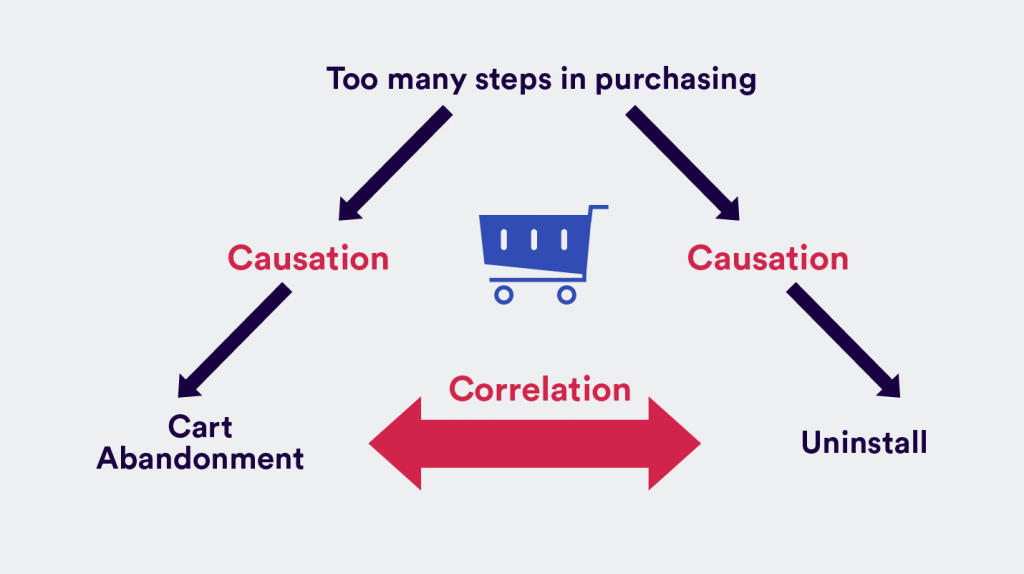For example, for the two variables “hours walked” and “weight reduced” there is a relationship between the two if the increase in hours walked is associated with an decrease in weight lost. If we consider the two variables “hours” worked and “salary” increases, as the price of wages increases a person’s ability to work will also increase (assuming a constant income).

Correlation is a statistical unit that describes the size and direction of a relationship between two or more variables. A correlation between variables, however, does not automatically mean that the change in a variable is the cause of the change in the values of another variable.

Causation denotes one event is the result of the phenomenon of the other event; i.e. there is a causal relationship between the 2 events. This is also referred to as cause and effect.

Theoretically, the difference between the two kinds of relationships are easy to determine — an action can cause another (e.g. smoking causes an increase in a risk of developing a lung cancer), or it can correlate with another (e.g. smoking can be correlated with alcoholism, but it does not actually cause alcoholism). In practice, however, it remains tough to clearly establish cause and effect, compared with establishing correlation.

### ♦Why are correlation and causation important?

The reason of much research or scientific analysis is to determine the extent to which a variable relates to another variable. For example:

• Is there a relationship between a person’s education level and his health?
• Is ownership of a pet associated with living longer?
• Did a company’s marketing campaign will increase their product sales?

These and some other questions are making understand whether a correlation exists between the 2 variables, and if there is a correlation then this may guide further research into determining whether one action causes the other.

### ♦How is correlation measured?

For two variables, a statistical correlation is determined by the use of a Correlation Coefficient, represented by the symbol (r), that is a single number that indicates the degree of relationship between two variables.

The coefficient’s numerical value ranges from (+1.0 to –1.0), which provides a description of the strength and direction of the relationship.

If the correlation coefficient consists of a negative value i.e. below 0, it will display a negative relationship between the variables. This means that the variables move in opposite direction.

If the correlation coefficient has a positive value (above 0) it indicates a positive relationship between the variables meaning that both variables move in tandem, i.e. as one variable decreases the other also decreases, or when one variable increases the other also increases.

Where the correlation coefficient is 0 this indicates there is no relationship between the variables (one variable can remain constant while the other increases or decreases).

While the correlation coefficient is a useful measure, it has its limitations:

Correlation coefficients are usually associated with measuring a linear relationship.

For example, if you compare hours worked and income earned for a tradesperson who charges an hourly rate for their work, there is a linear (or straight line) relationship since with each additional hour worked the income will increase by a consistent amount.

If, however, the tradesperson charges based on an initial call out fee and an hourly fee which progressively decreases the longer the job goes for, the relationship between hours worked and income would be non-linear, where the correlation coefficient may be closer to 0.

Care is needed when interpreting the value of ‘r’. It is possible to find correlations between many variables; however, the relationships can be due to other factors and have nothing to do with the two variables being considered.

For example, sale of an ice cream candy and the sale of  a sunscreen lotion can increase and decrease though out a year in a systematic pattern, but it can be a relationship that can be due to the effects of the season (i.e. hotter the weather sees an increase in people wearing sunscreen lotion as well as eating an ice cream candy) rather than due to any direct relationship between sales of sunscreen and ice cream.

The correlation coefficient must not be used to say anything about cause and effect relationship. By examining the value of ‘r’, we can conclude that two variables are related, but that ‘r’ value does not indicate if one variable was the cause of the change in the other.

### ♦How can causation be established?

Causality is the area of statistics that is generally misunderstood and misused by people in their mistaken belief that because the data show a correlation than there is definitely an underlying causal relationship.

The use of a controlled study is the most effective pattern of creating causality between two variables. In a controlled study, the sample or population is split into two parts, with both groups being comparable in almost every scenario. The two groups then receive different treatment processes, and the outcomes of each group are examined.

Example:

In medical research, one group might be given a placebo while the other group is given a new type of medication. If the two groups have determinable different outcomes, the different experiences may have caused the different outcomes.

Due to ethical reasons, there are limits to the use of controlled studies; it would not be appropriate to use two comparable groups and have one of them undergo a harmful activity while the other does not. To overcome this situation, observational studies are often used to investigate correlation and causation for the population of interest. The studies can look at the groups’ behaviors and outcomes and observe any changes over time.

The objective of these studies is to display statistical information to add to some other sources of information that would be required for a process of establishing whether or not causality exists between the two variables.

## Chi Square test

What Is a Chi Square test?

• A chi square (χ2) test is a test that measures how expectations are compared to actually observed data.
• The data used in calculating a chi square test must be random, raw, mutually exclusive, drawn from independent variables, and drawn from a large enough sample.
• It is often used in hypothesis testing.
• Example: The results of tossing a coin 1000 times meet these criteria

Why we need Chi Square test?

• There are two main types of chi square tests:
• The test of independence, that asks a question of relationship, like, “Is there a relationship between gender and SAT scores?”
• The goodness-of-fit test, that asks something like “If a coin is tossed 1000 times, will it be heads 500 times and tails 500 times?”
• For these kind of tests, degrees of freedom are used to identify if a specific null hypothesis can be rejected based on the total number of variables and samples taken in the experiment.
• For example, Consider, employees and their vehicle chosen to travel home, a sample size of 30 or 40 employee’s is likely not large enough to create significant amount data. Getting the same or similar results from a study using a sample size of 400 or 500 employees is more valid.

How to Calculate Chi Square test?

Formula: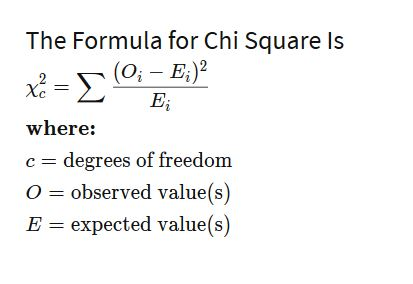Ex)  Imagine a random poll was taken across 20,000 different voters, both male and female. The people who responded were classified according to their gender and whether they were republican, democrat or an independent.

Imagine a grid with the columns labeled republican, democrat, and independent, and two rows labeled as male and female. Assume the data from the 20,000 respondents is as follows:

 Republican Democrat Independent Total Male 4000 3000 1000 8000 Female 5000 6000 1000 12000 Total 9000 9000 2000 20000

• Step1) Find the expected frequencies.

These are calculated for each “cell” in the grid. As such there are two categories of gender and three categories of political view, there are total six expected frequencies. The formula for the expected frequency is: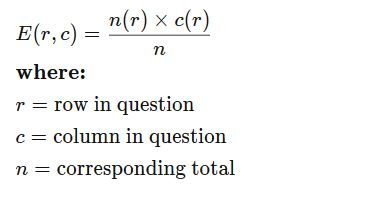Hence:

• E(1,1) = (9000*8000)/20000 =3600
• E(1,2) =(9000*8000)/20000 =3600
• E(1,3) =(2000*8000)/20000 =800
• E(2,1) =(9000*12000)/20000 =5400
• E(2,2) =(9000*12000)/20000 =5400
• E(2,3) =(2000*12000)/20000 =1200

Step2) these values are the used to calculate the chi squared statistic using the following formula: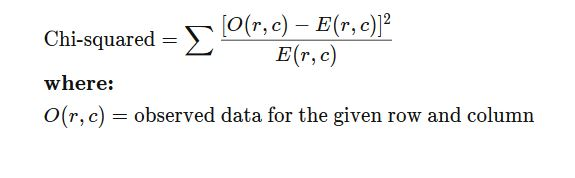• O(1,1)=(4000-3600)²/3600 = 44.44
• O(1,2)=(3000-3600)²/3600 = 100
• O(1,3)=(1000-800)²/800 = 50
• O(2,1)=(5000-5400)²/5400 = 29.63
• O(2,2)=(6000-5400)²/5400 = 66.66
• O(2,3)=(1000-1200)²/1200 = 33.33

Chi-squared = 324.66

The chi squared statistic then equals to the sum of these value, or 324.66. We can then look at a chi squared statistic table to see, given the degrees of freedom in our set-up, whether the result is statistically significant or not.

## Squared error of regression line

### ♦What is Regression Line?

Regression is a statistical measurement used in finance, investing attempts to identify the strength of the relationship between a dependent variable (usually denoted by Y) and a series of other changing variables (known as independent variables).

Regression helps investors and financial managers to value assets and understand the relationships between variables, such as commodity prices and the stocks of businesses dealing in those commodities.

There are two basic types of regressions:

►Linear Regression.

►Multiple Linear Regression.

Also there are some non-linear regression methods for more complicated data and analysis.

Linear regression uses an independent variable to predict the outcome of the dependent variable Y, while multiple regression uses two or more independent variables to predict the outcome.

### ♦Why we use Regression?

Regression can help financer’s and investment professionals as well as professionals in other businesses.

Regression can also help to predict sales for an organization based on weather, previous sales, GDP growth or other types of conditions. The capital asset pricing model often uses regression model in finance for pricing assets and discovering costs of capital.

Regression takes a group of some random variables, thought to be predicting Y, and tries to determine a mathematical relationship between them. This relationship is in the form of a straight line (linear regression) that best approximates all the individual data points.

In multiple regression, the separate variables are differentiated by using numbers with subscripts.

### ♦How to calculate Regression?

The general form of each type of regression is:

Linear regression: Y = a + bX + u

Multiple regression: Y = a + b1X1 + b2X2 + b3X4 + … + btXt + u

►Where:

Y = the variable that you are trying to predict (dependent variable).

X = the variable that you are using to predict Y (independent variable).

a = the intercept.

b = the slope.

u = the regression residual.

### ♦What does the Mean Squared Error Tell You?

The mean squared error shows you how close a regression line is to a set of points. It does this by taking distances from the points to the regression line (these distances are actually the “errors”) and squaring them. The squaring is necessary in-order to remove any negative signs. It also gives more weight to larger differences. It’s known as the mean squared error as you’re finding the average of a set of errors.

 Height 43 44 45 46 47 Weight 41 45 49 47 44

Find Mean Squared Error?

►Step 1: Find the regression line.

y= 9.2 + 0.8x.

►Step 2: Find the new Y’ values:
9.2 + 0.8(43) = 43.6
9.2 + 0.8(44) = 44.4
9.2 + 0.8(45) = 45.2
9.2 + 0.8(46) = 46
9.2 + 0.8(47) = 46.8

►Step 3: Find the error (Y – Y’):
41 – 43.6 = -2.6
45 – 44.4 = 0.6
49 – 45.2 = 3.8
47 – 46 = 1
44 – 46.8 = -2.8

►Step 4: Square the Errors:
-2.62 = 6.76
0.62 = 0.36
3.82 = 14.44
12 = 1
-2.82 = 7.84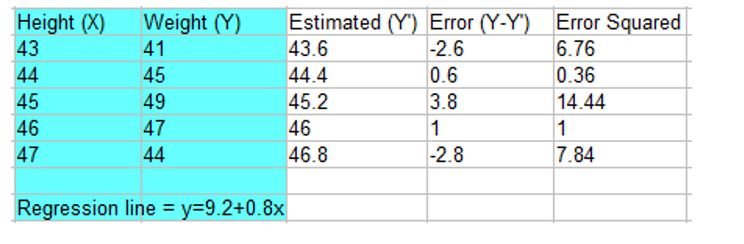►Step 5: Add all of the squared errors up: 6.76 + 0.36 + 14.44 + 1 + 7.84 = 30.4.

►Step 6: Find the mean squared error:
30.4 / 5 = 6.08.

## Type 1 error and Type 2 error

### ♦What is Type 1 error?

A type I error is a type of error that occurs during a hypothesis testing process when a null hypothesis is rejected even though it is true and should not be rejected.

In hypothesis testing, a null hypothesis is established before starting a test.

In some cases, the null hypothesis assumes the absence of a cause and effect relationship between the items being tested and the inducement being applied to the test subject in pursuance of triggering an outcome to the test.

This is denoted as “n=0”.

When the test is conducted, the result seems to denote the inducement applied to the test subject will cause a reaction then the null hypothesis that the inducement has no effect on the test subject will get rejected.

### ♦Why we use Type 1 error?

Sometimes, rejecting a null hypothesis that there is no relationship between the test subject, the inducement and the outcome can be incorrect.

If the result of the test is caused by something other than the inducement, it can cause a “false positive” outcome where it appears the inducement acted upon the subject, but the outcome was actually caused by chance. This “false positive,” leading to an incorrect rejection of the null hypothesis, is called a type I error. A type I error rejects an idea that must not have been rejected.

Example of a Type I Error

Example,

Let’s consider the trail of an accused criminal.

The alternate hypothesis is that the person is guilty, while the null Hypothesis is innocent.

A Type I error in this case would be that the person is not found innocent and is sent to jail, in spite of actually being innocent.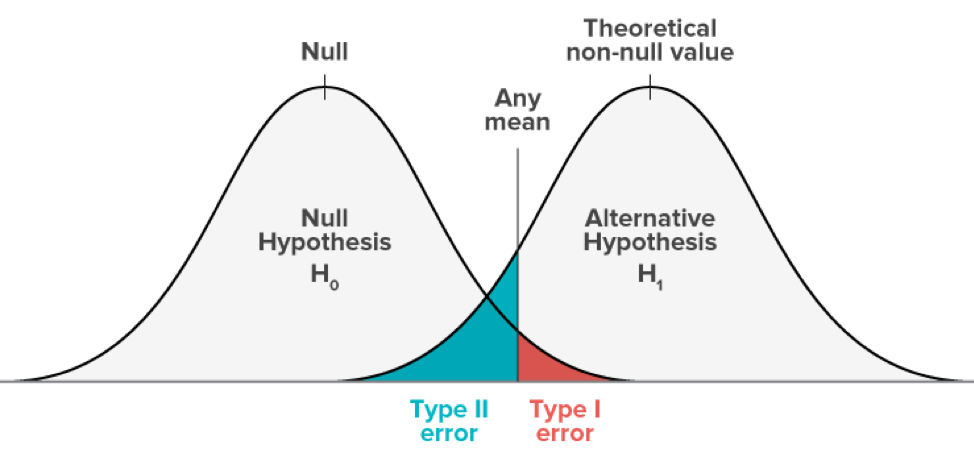### ♦What is Type II error?

A type II error is a part of Hypothesis testing that describes the error, which occurs when one fails to reject a null hypothesis that is actually false.

In other words, it produces a false positive. The error rejects the alternative hypothesis, even after it does not occur due to chance.

Why we need Type II error?

Consider a biotechnology organization wants to compare how effective two of its drugs are for treating cancer.

The null hypothesis states the two medicines are equally effective.

A null hypothesis (Ho), claims that the organization hopes to reject using the one-tailed test.

The alternative hypothesis (Hα), states that, two drugs are not equally effective. The alternative hypothesis, Hα, is the measurement supported by rejecting the null hypothesis.

The biotech organization implements a large clinical trial of 9,000 patients with cancer to compare the treatments. The company expects, two drugs must have an equal number of patients to determine that both drugs are effective. It selects a significance level of 0.05, which indicates it is willing to accept a 5% chance it may reject the null hypothesis when it is true or a 5% chance of committing a type I error.

Assume beta is calculated to be 2.5%. Hence, the probability of committing a type II error is 2.5%. If the two medications are not equally effective, the null hypothesis should be rejected.

However, if the biotech organization does not reject the null hypothesis when the drugs are not equally effective, a type II error occurs.

## T-Statistics

### ♦What is T-statistics?

The T-Statistic is required in a T test when you are in position to decide if you should accept or reject the null hypothesis.

It’s somewhat similar to a Z-score and you use it in the same way, i.e. to find a cutoff point or to find your t score or to compare the two.

You use the T-statistic when you have a small sample size, or if you don’t have the population standard deviation.

The T statistic does not really tell you much on its own. It’s just like a word “sum” doesn’t mean anything on its own, without some context. If I say “the sum was 550000,” it means nothing.

But if I say “the sum of salaries of employees is Rs.550000” in an organization then the picture becomes clearer. In the same way, you need some more information along with your t-statistic for it to make some sense. You get this information by taking a sample or by running a hypothesis test.

### ♦Why we need T Statistic?

When you implement a hypothesis test, you use the T statistic with a p-value.

The p-value indicates what the odds are that your results could have happened by chance. Let’s consider you with some of your friends score an average of 215 on a bowling game. You know the average bowler scores 79.9.

So in order to decide that you and your friends should consider professional bowling? Or are those scores a fluke? Finding the t-statistic and the probability value will give you a good idea. To be more technical, finding those values gives you evidence of a significant difference between your team’s mean and the population mean (i.e. everyone).

The greater the value of T, the more evidence you have that your team’s scores are significantly different from average.

Smaller the value of T value is evidence that your team’s score is not significantly different from average. It’s pretty obvious that your team’s score (215) is significantly different from 79.7, so you’d want to take a look at the probability value.

If the p-value is larger than 0.05, the odds are your team getting those scores are due to chance. Very small (under 0.05), you’re onto something: think about becoming a professional.

### ♦T Score vs. Z-Score.

The Z-score allows you to decide whether your sample is different from the population mean. In order to use z-score, you must know four things:

The population mean.

The population standard deviation.

The sample mean.

The sample size.

Mostly in statistics, you don’t have any knowledge about a population, so instead of a Z score we use a T Test with a T Statistic. The major difference between using a Z score and a T statistic is that you need to estimate the population standard deviation.

The T test is also used when you have a small sample size (less than 30).

### ♦How to calculate T-test?

Formula

The T-statistic can be found in so many different ways, there is no single formula for it. It depends on what type of test you are trying to do.

For example:

One sample t-test. This is one of the most common type of t test which you’ll come across in elementary statistics. You can test the mean of a single group against a known mean. For example, take the average IQ as 100. You can examine a class of students with a mean score of 90 to see if that’s significant, or if it occurs by chance.

A paired t-test compare means from the same group at different times (say, one year apart). Example, you can try a new weight loss technique on a group of people and follow up a year later.

### Quick Support